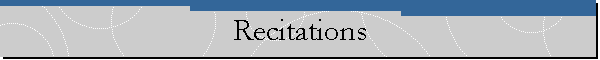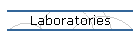Analytical Schedule of Recitation1st week Topics: Using computer algebra packages (Mathematica, Matlab, MathCad) Using an electric circuit simulator (SPICE) Basic Points: Examples: Representative examples from the topic of the week.   Applications: 2nd week Topics: Methodology: Basic Points: Understand the concept of transfer function  Be familiar with the decibel (dB) scale  Learn how to make Bode magnitude and phase plots  Learn about series and parallel resonant RLC circuits  Know different types of passive and active filters and their characteristics  Understand the use of scaling in circuit analysis  Be able to use PSpice to obtain frequency response  Examples: Representative examples from the topic of the week.   Applications: Apply what is learnt to radio receiver and touch-tone telephone 3rd week Topics:   Methodology:   Basic Points: Know the definition of the Laplace transform  Be familiar with the properties of the Laplace transform  Be able to find the inverse Laplace transform  Learn the concept of convolution  Examples: Representative examples from the topic of the week.   Applications: Apply what is learnt to integrodifferential equations 4th week Topics: Methodology: Basic Points: Learn how to apply the Laplace transformation techniques to circuit analysis  Understand to how to Laplace transform circuit elements  Learn how to find the transfer function  Learn how to apply state variables to analyze circuits with several inputs and outputs  Apply what is learnt to network stability and network synthesis  Examples: Representative examples from the topic of the week.   Applications:  Be able to use MATLAB to find system response 5th week Topics: Methodology: Basic Points: Examples: Representative examples from the topic of the week.   Applications: 6th week Topics: Methodology: Basic Points: Examples: Representative examples from the topic of the week.   Applications: 7th week Topics: Methodology: Basic Points: Examples: Representative examples from the topic of the week.   Applications: 8th week Topics: Methodology: Basic Points: Examples: Representative examples from the topic of the week.   Applications: 9th week Topics: Methodology: Basic Points: Examples: Representative examples from the topic of the week.   Applications: 10th week Topics: Methodology: Basic Points: Learn about two-port networks  Be familiar with the z, y, and h parameters and their relationships  Learn about interconnecting two-ports  Be able to use PSpice to compute two-port parameters  Examples: Representative examples from the topic of the week.   Applications:  Apply what is learnt to transistor circuits and ladder network synthesis 11th week Topics: Methodology: Basic Points: Know the definition of Fourier series  Learn how to find the Fourier series of a signal  Be able to take advantage of symmetry in finding Fourier series  Apply Fourier series to circuit analysis  Learn about average power and rms values  Learn about exponential Fourier series  Be able to use PSpice to do Fourier analysis  Examples: Representative examples from the topic of the week.   Applications:  Apply what is learnt to spectrum analyzers and filters 12th week Topics: Methodology: Basic Points: Understand magnetically coupled circuits  Learn the concept of mutual inductance  Be able to determine energy in a coupled circuit  Learn how to analyze circuits involving linear and ideal transformers  Be familiar with ideal autotransformers  Learn how to analyze circuits involving three-phase transformers  Be able to use PSpice to analyze magnetically coupled circuits  Examples: Representative examples from the topic of the week.   Applications: Apply what is learnt to transformer as an isolation device and power distribution 13th week Topics: Methodology: Basic Points: Know the definition of Fourier transform  Learn the properties of Fourier transform  Learn to apply Fourier transform in analyzing circuits  Understand Parsevals theorem  Examples: Representative examples from the topic of the week.   Applications: Apply what is learnt to amplitude modulation (AM) and sampling 14th week Review: Topics: Methodology: Basic Points: Examples: Representative examples from the topic of the week.   Applications: 15th week Review: Topics: Methodology: Basic Points: Examples:   Representative examples from the topic of the week.   Applications: 16th week Final examination: